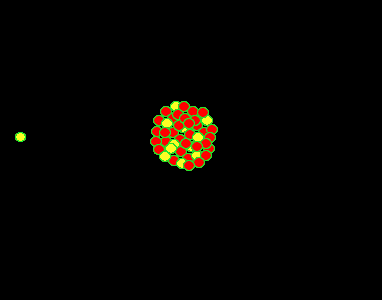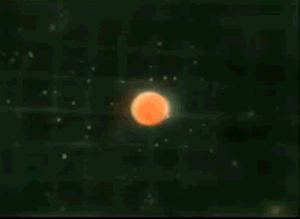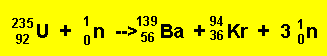Nuclear energy Fission reaction E = mc2 Above is Einstein's famous equation relating mass and energy. Where: E is the energy released m = is the mass c = speed of light.It was found, by Lise Meitner, that the uranium (235U) atom can be split into two lighter, more stable, smaller nuclei by the firing of a single neutron into its nucleus. When the neutron hits, it causes the nucleus to oscillate straining the nuclear force and eventually splitting the nucleus. In this fission process the mass of the uranium atom is heavier than the combined mass of the products.This small mass difference is converted into energy according to the equation E=mc2. Now even a small mass multiplied by the speed of light gives a large amount of energy. Now multiply this by the number of uranium atoms in a kilogram of uranium and the amount of energy released from a kilogram of uranium is breathtaking. This is why nuclear weapons are so devastating when compared to conventional (TNT) weapons. The nuclear fission reaction can be summed up by the equation below.Note how the number of nucleons is the same on both sides of the equation, 236 nucleons on the left and 236 on the right. So where does the energy come from? The total mass of the nucleons on the left is slightly more than the nucleons on the right. This tiny mass difference is converted into energy (heat and light) via the relationship E=mc2 Continue

Home